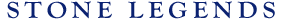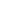HOMEPRODUCTSIDEA CENTERSERVICESTECHNICALABOUT USHello Guest Login  |  Become Member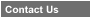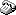800-398-1199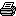214-398-1293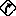Map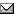Email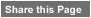Click to Share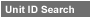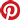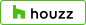Select which variables you would like to search by.Type DescriptionPrimary ViewPrimary ModifierUnit NameView Definitions

View Definitions

View DefinitionsX-Dim Y-Dim Z-Dim XX-Dim YY-Dim ZZ-Dim R-Dim D-Dim P-Dim
 Click column heading to re-sort results list.Product Name Primary View Modifier Pri Dimx  y  z Alt Dimxx  yy  zz Alt2 Dimr  d  p E\$timated Thumbnail Alpine Table Base1 Pedestal SpecialShape Stacked X = 27" Y = 28"Z = 27" XX = n/a YY = n/aZZ = n/a R = n/a D = 27"P = n/a \$ 475.00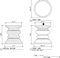Alpine Table2 Pedestal SpecialShape X = 39" Y = 28"Z = 39" XX = n/a YY = n/aZZ = n/a R = n/a D = 39"P = n/a \$ 735.00Arabella Escort Pedestal SpecialShape X = 20" Y = 50"Z = 21" XX = n/a YY = n/aZZ = n/a R = n/a D = n/aP = n/a \$ 462.00Archer Escort Pedestal Octagonal X = 10" Y = 34-1/2"Z = 24-1/2" XX = n/a YY = 32"ZZ = n/a R = n/a D = n/aP = 22" \$ 163.20Bullnose Chamfer6 Pedestal Rectangular X = 52-1/2" Y = 29-1/8"Z = 27-1/2" XX = n/a YY = n/aZZ = n/a R = n/a D = n/aP = n/a \$ 387.05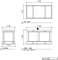Camelon Harp Pedestal Rectangular Ornament X = 33" Y = 35-9/16"Z = 21-9/16" XX = n/a YY = n/aZZ = 20-3/4" R = n/a D = n/aP = n/a \$ 385.12Carriage Escort Pedestal Square X = 14" Y = 34"Z = 14" XX = n/a YY = n/aZZ = n/a R = n/a D = n/aP = n/a \$ 159.94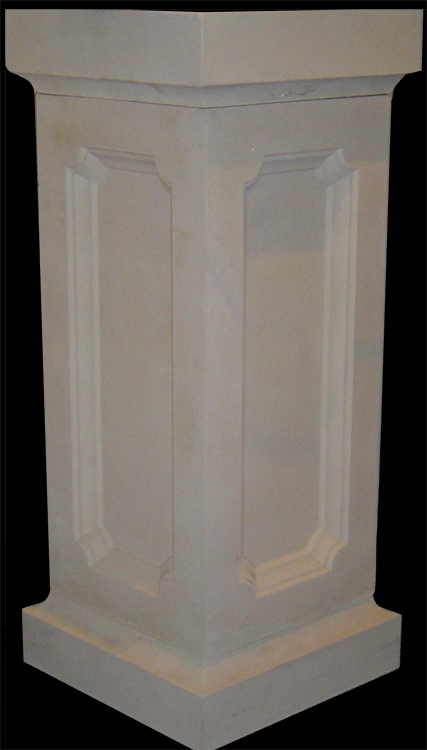Catori Pedestal Cylindrical Art X = 10" Y = 18"Z = 10" XX = n/a YY = n/aZZ = n/a R = n/a D = 10"P = n/a \$ 350.00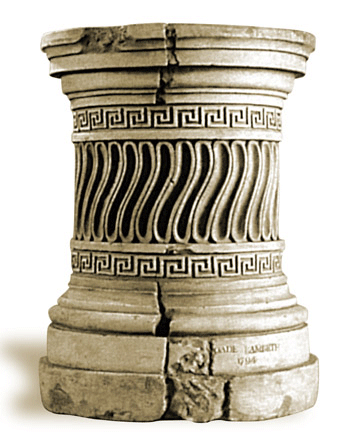Cavalon Pedestal Square Ornament X = 22-5/8" Y = 35-3/8"Z = 22-5/8" XX = n/a YY = n/aZZ = 22-5/16" R = n/a D = n/aP = n/a \$ 385.12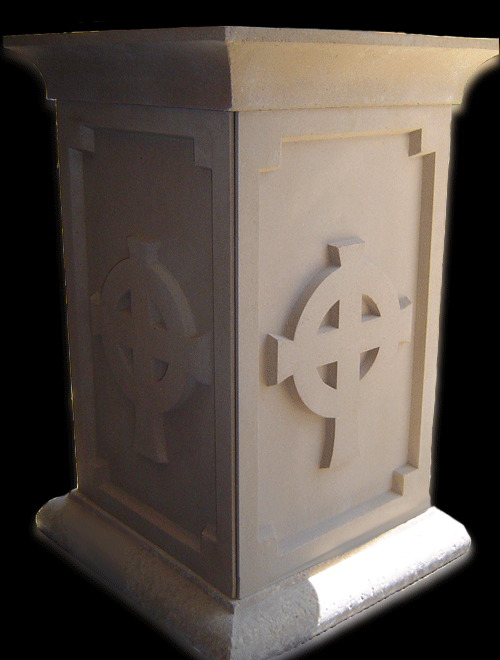Clarion Trifoil Pedestal Square Ornament X = 21-1/16" Y = 37-1/8"Z = 21-1/16" XX = n/a YY = n/aZZ = 20-3/4" R = n/a D = n/aP = n/a \$ 385.12Clover 1 Escort Pedestal Square X = 29-3/4" Y = 25-1/2"Z = 25-1/2" XX = n/a YY = n/aZZ = 20-7/16" R = n/a D = n/aP = n/a \$ 598.00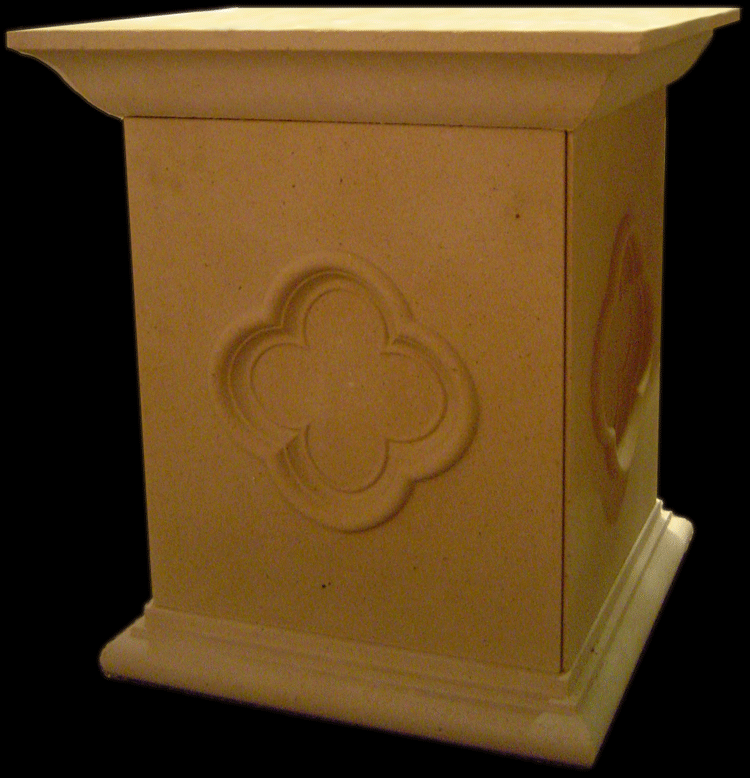Columbine Pedestal Pedestal Square Stacked X = 16-3/4" Y = 20-3/4"Z = 16-3/4" XX = n/a YY = n/aZZ = 14-5/8" R = n/a D = n/aP = 16-3/4" \$ 143.68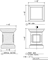Corinthian Cap 1 Pedestal SpecialShape X = 29-1/4" Y = 26-5/8"Z = 29-1/4" XX = n/a YY = n/aZZ = n/a R = n/a D = 18"P = n/a \$ 500.00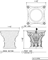Corinthian Cap 2 Pedestal SpecialShape X = 29-5/8" Y = 32-1/2"Z = 32-1/2" XX = n/a YY = n/aZZ = n/a R = n/a D = 20"P = n/a \$ 575.00Corinthian Pedestal Candle Holder Pedestal SpecialShape X = 3" Y = 17-1/2"Z = 3" XX = n/a YY = n/aZZ = n/a R = n/a D = 3"P = n/a \$ 49.61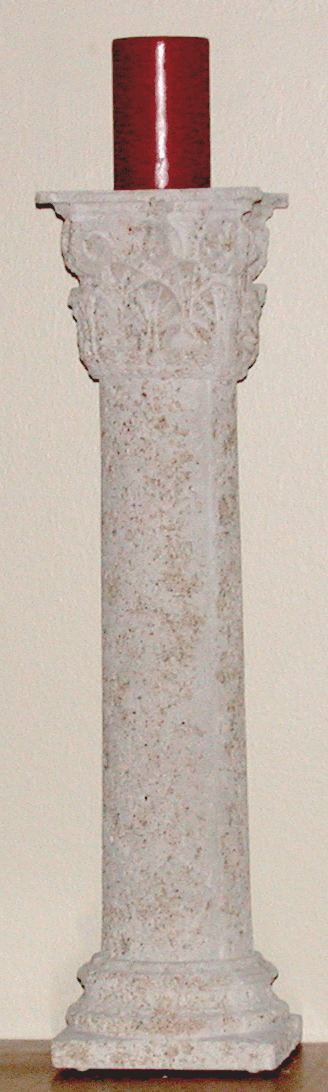Covina1 Pedestal Pedestal Square Solid X = 15-1/4" Y = 13-5/8"Z = 15-1/4" XX = n/a YY = n/aZZ = n/a R = n/a D = n/aP = n/a \$ 125.00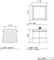Covina2 Pedestal Pedestal Square Solid X = 15-1/4" Y = 18"Z = 15-1/4" XX = n/a YY = n/aZZ = n/a R = n/a D = n/aP = n/a \$ 155.00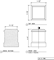Doric Pedestal Pedestal SpecialShape X = 22-1/2" Y = 22-1/2"Z = n/a XX = n/a YY = n/aZZ = n/a R = n/a D = 6-3/16"P = n/a \$ 137.93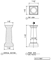Dualistique Pedestal SpecialShape X = 29-3/4" Y = 28-7/16"Z = 10-1/2" XX = n/a YY = n/aZZ = n/a R = n/a D = n/aP = 8" \$ 168.20Extrados19 Carriage Pedestal Square X = n/a Y = 34"Z = n/a XX = n/a YY = n/aZZ = n/a R = n/a D = n/aP = n/a \$ 336.72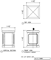Lion Rampant Escort Pedestal Square Art X = 19-3/4" Y = 36-3/4"Z = 19-3/4" XX = n/a YY = n/aZZ = 20-1/4" R = n/a D = n/aP = n/a \$ 389.92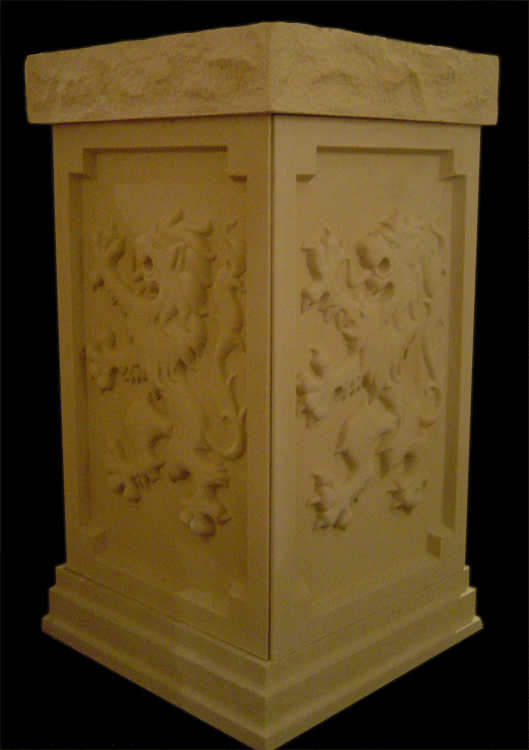Maynard Pedestal 1 Pedestal Circular X = 32-3/8" Y = n/aZ = n/a XX = n/a YY = n/aZZ = n/a R = n/a D = 55"P = n/a \$ 563.09Montgomery Pedestal Rectangular X = 20" Y = 42-5/16"Z = 20" XX = n/a YY = 33-9/16"ZZ = 18" R = n/a D = n/aP = n/a \$ 338.00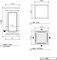Orion Colyer Pedestal Square 360Degree X = 20-1/2" Y = 29-3/4"Z = 20-1/2" XX = n/a YY = n/aZZ = n/a R = n/a D = n/aP = n/a \$ 275.05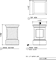Orpheus Escort Pedestal Octagonal Hollow X = 13-15/16" Y = 29-9/16"Z = 13-15/16" XX = n/a YY = n/aZZ = n/a R = n/a D = n/aP = n/a \$ 152.88Pandora Pedestal 1 Pedestal Rectangular X = 32" Y = 46"Z = 6-1/2" XX = n/a YY = n/aZZ = 4" R = n/a D = n/aP = n/a \$ 579.84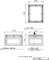Pandora Pedestal 2 Pedestal Rectangular 360Degree X = 35-1/4" Y = 48"Z = 7" XX = 48" YY = 60"ZZ = 4" R = n/a D = n/aP = n/a \$ 771.12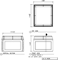Recess Pedestal Pedestal X = n/a Y = n/aZ = n/a XX = n/a YY = n/aZZ = n/a R = n/a D = n/aP = n/a \$ 295.45Renata Castile Pedestal Square X = 22-5/8" Y = 35-3/8"Z = 22-5/8" XX = n/a YY = n/aZZ = 22-5/16" R = n/a D = n/aP = n/a \$ 370.30Sophie's Crush Escort Pedestal Rectangular X = 36" Y = 34-5/16"Z = 25-13/16" XX = n/a YY = n/aZZ = n/a R = n/a D = n/aP = n/a \$ 512.00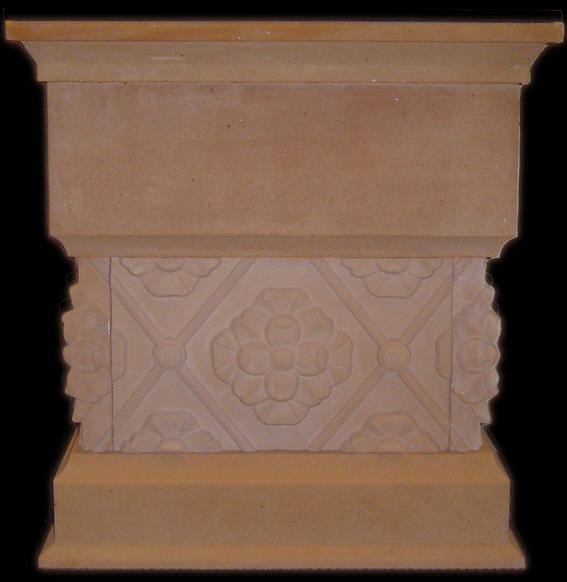Tuscan Pedestal Pedestal Square X = " Y = "Z = " XX = " YY = "ZZ = " R = " D = "P = " \$ 222.35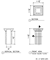VeronaPedestal Pedestal Circular X = 17" Y = 28-1/4"Z = 17" XX = n/a YY = n/aZZ = n/a R = n/a D = 17"P = n/a \$ 320.00VeronaRope Pedestal Rope X = 11-1/4" Y = 36"Z = 11-1/4" XX = n/a YY = n/aZZ = n/a R = n/a D = 11-1/4"P = n/a \$ 199.64